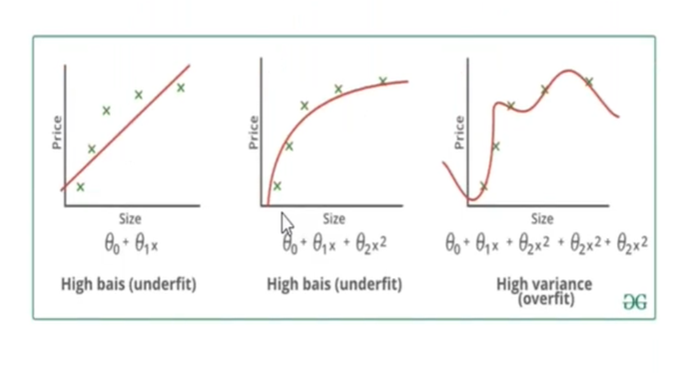top of pageSearch

Bias is an error introduced in the model due to oversimplification of the machine learning algorithm. It can lead to underfitting. High bias can cause an algorithm to miss the relevant relations between features and target outputs

Variance is error introduced in the model due to complex machine learning algorithm, the model learns noise also from the training dataset and performs badly on test dataset. It can lead to high sensitivity and overfitting.

The goal of any supervised machine learning algorithm is to have low bias and low variance to achieve good prediction performance. More often than not, we are challenged with one of the below scenarios as there is no escaping the relationship between bias and variance in machine learning. Increasing the bias will decrease the variance. Increasing the variance will decrease bias.

· If there is a high variance into values in a dataset, then the model will learn the dataset but it will not predict the values properly.

· If there is a high variance and low bias, the model predicted values are around or close to the target.

· If there is low variance and low bias, the model predicted values will be right on the spot

· If there is low variance and high bias, the predicted values will start hitting somewhere and not on the target. However, the values are at the same region.

· If there is high variance and high bias, the predicted values will be somewhere else away from the targeted values

In the figure below, it can be noticed in the first graph is a high bias, high variance case as the predicted values are away from trend line. Due to the high bias the model will not learn anything as it skips all the values causing underfitting. From the third graph it is evident the model it going to learn everything which is not desirable for better model performance and hence deteriorating the model performance.Underfitting and overfitting arises both in regression and classification as well.

Regularization

There is a method to overcome the bias, called Regularization. This technique introduces a cost term for bringing in more features with the objective function. Hence it tries to push the coefficients for many variables to zero and reduce cost term. This helps to reduce model complexity so that the model can become better at predicting.

There are two kinds of regularization techniques. Lasso regression(L1) and Ridge Regression(L2). These techniques try to reduce the features that are not important. In case of Lasso regression, you have coefficients of different different columns, some are adding and some are negating. now, this lasso algorithm will internally detect which of the column is impacting highly the output variable and which column is not. It will internally make some of these column coefficients to ‘0’.

With Ridge regression, when there are column coefficient values and there are high variance coefficients, it will internally try to reduce the variance of the columns

Let us understand regularization through Boston housing prices dataset. First, perform linear regression on the dataset. Apply Lasso regression and Ridge regression on the model, then compare the model performance before and after regularization.Now, we will try to minimize coefficient variance by applying Ridge regression.Now compare the model performances by estimating Lasso and Ridge regression model metrices such as MAE,MSE,RSE and RMSE.